## Reservoir Properties and Completion Selections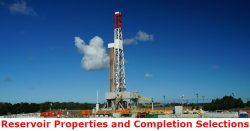In order to properly design a completion, reservoir rock and fluid properties must be carefully taken into account because they directly influence on equipment selection.  Reservoir properties (rock and fluid properties) which must be considered are as follows;

## Rock Properties

Permeability (k)

Low permeability formation may require fracturing operation to enhance production. The completion for tight formations must be able to withstand pumping pressure and allow fracking fluid and proppant to flow through.

Formation Strength

Unconsolidated formations are required to complete a well with a sand control completion; thus, a well can be produced without any damage to downhole and surface equipment.

Formation Pressure

Reservoir pressure directly affects the pressure rating on all completions because all components must be able to work under reservoir condition. What’s more, formation pressure will affect how much flow of the well can produce.

Formation Temperature

High reservoir temperature will quickly degrade some components, especially elastomer, and this will result in well integrity issues due to pressure leakage. This is one of the critical concerns in selecting the right equipment to work under high temperature conditions. Continue reading

## Determine Compressibility Factor with Present of CO2 and H2SThis example will demonstrate how to determine the compressibility factor (z) for gas with CO2 and H2S. As it is described in the article,Determine Compressibility of Gases , it states that gas with CO2 and H2S must be corrected. This is similar to a normal method for determining z-factor but it is required some correction. Please follow the steps below;

Gas component is shown in Table 1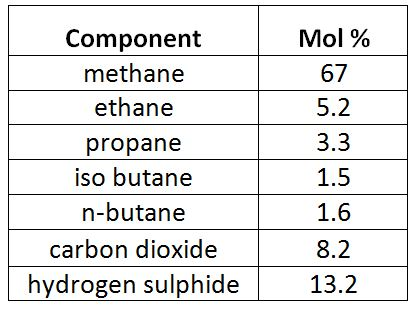Table 1 – Gas Component

Reservoir pressure = 6,000 psia

Reservoir temperature = 190 F Continue reading

## Example of Real Gas Calculation

This example will demonstrate how to calculate the compressibility of real gas in order to determine gas density and specific gravity at a specific condition.

Calculate the following based on the given condition:

1) Density of this gas under the reservoir conditions of 7,500psia and 220ºF,

2) Specific gravity of the gas.

Gas component is shown in Table 1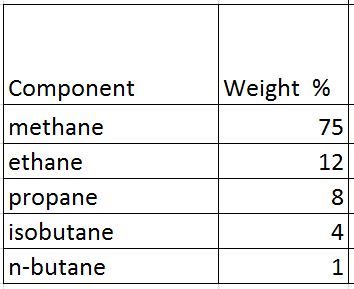Table 1 – Gas Component

Average density of air = 28.96 lb/cu-ft

## Solution

• Determine critical pressure and temperature of gas mixtures using Kay’s ruleTable 2 – Critical Pressure and Temperature

Note: critical pressure and temperature can be found from this link – https://www.drillingformulas.com/determine-compressibility-of-gases/

Pc’ = Σyipci = 660.5 psia

Tc’ = ΣyiTci = -46.2 F = -46.2 +460 F = 413.8 R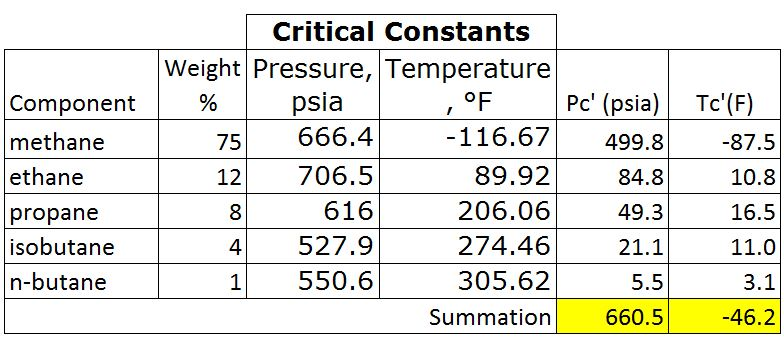Table 3 – Pc’ and Tr’ by Kay’s Rule

• Calculate Tr and Pr

Tr = T ÷Tc

Tr = (220+460) ÷ (-46.2+460)

Tr = 1.64

Note: temperature must be in Rankin.

Rankin = Fahrenheit + 460

For the critical temperature calculation, it can be converted the critical temperature from F to R before calculating Tc’. This will still give the same result.

Pr = P ÷ Pc

Pr = 7500 ÷ 660.5 = 11.4

• Read the compressibility factor (z) from the chart.

z = 1.22Figure 1-z-factor from the Standing and Katz Chart

• Calculate average molar mass

Average Molar Mass = Σyi×Mi = 22.1 lbTable 4 – Average Molar Mass of Gas

• Calculate density of gas from the equation below;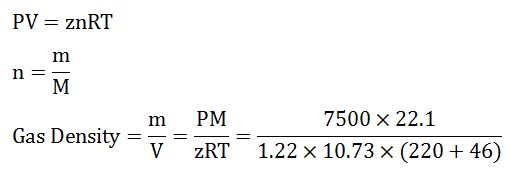Gas Density = 18.6 lb/cu-ft

• Calculate gas specific gravity from the equation below;

SG = Gas Density ÷ Air Density

SG = 18.6 ÷ 28.96

SG = 0.64

Summary:

Gas Density = 18.6 lb/cu-ft

SG = 0.64

We wish that this example will help you understand to determine z-factor and use it to calculate any related information.

References

Abhijit Y. Dandekar, 2013. Petroleum Reservoir Rock and Fluid Properties, Second Edition. 2 Edition. CRC Press.

L.P. Dake, 1983. Fundamentals of Reservoir Engineering, Volume 8 (Developments in Petroleum Science). New impression Edition. Elsevier Science.

Tarek Ahmed PhD PE, 2011. Advanced Reservoir Management and Engineering, Second Edition. 2 Edition. Gulf Professional Publishing.

## Determine Compressibility of Gases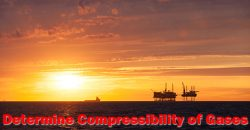This article will demonstrate how to determine gas compressibility by using simplified equation of state.

At a low pressure condition, gas similarly behaves like ideal gas and the equation for the ideal gas law is listed below;

# PV = nRT

Where;

P = pressure

V = gas volume

T = absolute temperature

n = number of moles of gas

R = gas constant

Gas constant (R) is different because it depends on the unit system used in the calculation. The R in the different unit system is demonstrated in the below table.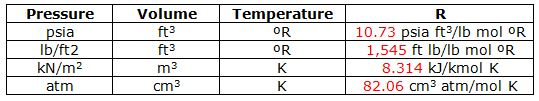Table 1 – Gas Constant (R) at Different Unit

## Volumetric Method To Estimate Volume In Place and ReservesVolumetric is a method to estimate fluid in reservoir based on volume of pore space in a rock and water saturation.

Volume of Oil Initially In Place (OIIP)

To estimate oil initially volume in place, the following formula is a volumetric calculation for oil.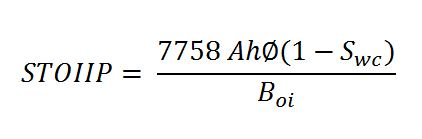Where;

STOIIP = stock tank oil in place, stb

A= area, acre

h = reservoir thickness, ft

ɸ = rock porosity, %

Swc =connate water saturation, %

Boi = oil formation volume factor, rb/stb

Note: the stock tank condition is a standard surface condition of oil and gas at 60F and 14.7 psia. Continue reading